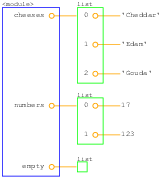# 14.3: Object DiagramsFigure $$\PageIndex{1}$$: Object diagram.

This example generates an object diagram showing the lists from Section 10.1. You can download it from http://thinkpython.com/code/lumpy_demo3.py.

from swampy.Lumpy import Lumpy

lumpy = Lumpy()
lumpy.make_reference()

cheeses = ['Cheddar', 'Edam', 'Gouda']
numbers = [17, 123]
empty = []

lumpy.object_diagram()


Figure 22.3.1 shows the result. Lists are represented by a box that shows the indices mapping to the elements. This representation is slightly misleading, since indices are not actually part of the list, but I think they make the diagram easier to read. The empty list is represented by an empty box.Figure $$\PageIndex{2}$$: Object diagram.

And here’s an example showing the dictionaries from Section 11.4. You can download it from http://thinkpython.com/code/lumpy_demo4.py.

from swampy.Lumpy import Lumpy

lumpy = Lumpy()
lumpy.make_reference()

hist = histogram('parrot')
inverse = invert_dict(hist)

lumpy.object_diagram()


Figure 22.3.2 shows the result. hist is a dictionary that maps from characters (single-letter strings) to integers; inverse maps from integers to lists of strings.Figure $$\PageIndex{3}$$: Object diagram.

This example generates an object diagram for Point and Rectangle objects, as in Section 15.6. You can download it from http://thinkpython.com/code/lumpy_demo5.py.

import copy
from swampy.Lumpy import Lumpy

lumpy = Lumpy()
lumpy.make_reference()

box = Rectangle()
box.width = 100.0
box.height = 200.0
box.corner = Point()
box.corner.x = 0.0
box.corner.y = 0.0

box2 = copy.copy(box)

lumpy.object_diagram()


Figure 22.3.3 shows the result. copy.copy make a shallow copy, so box and box2 have their own width and height, but they share the same embedded Point object. This kind of sharing is usually fine with immutable objects, but with mutable types, it is highly error-prone.